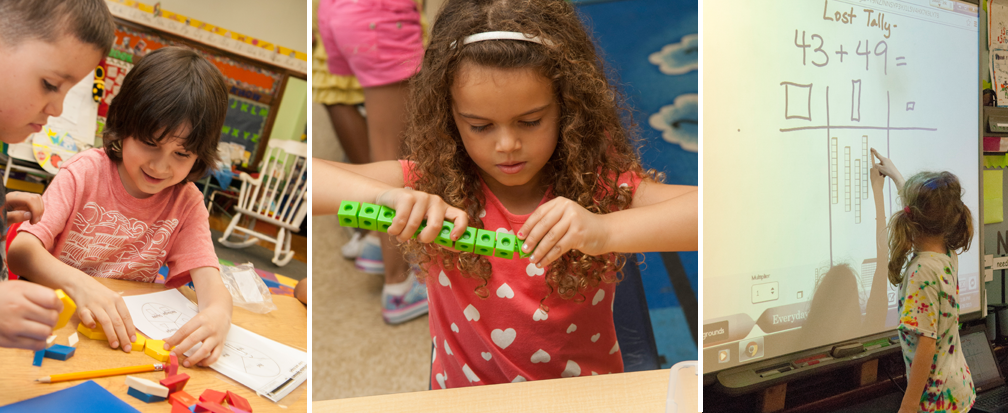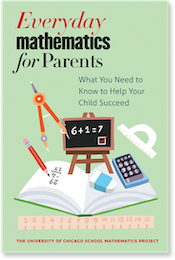## Alternative Algorithms

### What are the alternative algorithms? Why are these part of the Everyday Mathematics curriculum?

For decades, all American schoolchildren have been taught one standard procedure for each of the four basic operations of arithmetic. These "standard" algorithms, like the regrouping ("borrowing") algorithm for multi-digit subtraction and the long division algorithm, are not the only ways to perform these operations. There are many alternative algorithms taught in other countries. Compared to the standard U.S. algorithms, many of these alternative algorithms are more efficient and easier to learn.

Research has shown that teaching the standard U.S. algorithms fails with large numbers of children, and that alternative algorithms are often easier for children to understand and learn. For this reason, Everyday Mathematics introduces children to a variety of alternative procedures in addition to the customary algorithms. Examples of a couple alternative algorithms are below:

### Examples of Alternative Algorithms

#### Partial-Sums Algorithm

```                       2 6 8
+ 4 8 3
_______
6 0 0
1. Add 100s            1 4 0
4. Add partial sums    7 5 1```

#### Partial Product Algorithm

```          67
x 53
_______
50 x 60 3000
50 x 7   350
3 x 60   180
3 x 7     21
_______
3551```

Visit our Algorithms / Computation page for worked examples and practice exercises.The University of Chicago School Mathematics Project

University of Chicago Press